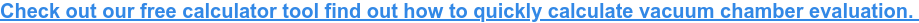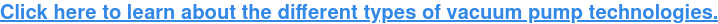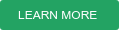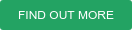For a vacuum pump system, a vital consideration in its design is the conductance. Conductance in vacuum systems is the characteristic of a vacuum component or system to readily allow the flow of gas and can be thought of as the inverse of resistance to flow. Its units are that of the volumetric capacity of gas flow in a passive component (or aggregate component of a vacuum system), such as an opening or a pipe, divided by time.

## Definition of Conductance

The conductance between two points is defined as the gas flow rate flowing through a device divided by the pressure drop that is driving it. Therefore, the dimensions that describe conductance are the same as pumping speed (volume per unit time) but conductance is a phenomenon usually used to describe piping and openings, not the vacuum pump.## how to determine the conductance influence

Conductance in a vacuum system is equivalent to conductance (inverse of resistance) in an electric circuit. Parallel conductance is the sum of each conductance, and series conductance is the inverse of the sum of each inverse conductance. If a system suffers from low conductance it can significantly reduce the effective pumping speed. This phenomenon becomes increasingly important as the pressure reduces and the flow transitions from a viscous regime to a molecular regime.

The conductance of a vacuum system needs to be calculated and incorporated into the system design and pump selection. Failure to do so can seriously impact how long the vacuum chamber will take to reach the desired pressure and may cause an ‘over-specification’ of a pump. A well-designed system will increase vacuum speed and efficiency, as well as minimise energy costs; the costs of failing to take conductance into account can be severe, these include delayed equipment start-up, plant downtime and process inefficiency.

Discover how the different components of a vacuum system impact conductance in our Ask Dr Chew series.## CONDUCTANCE IMPACT IN DIFFERENCE PRESSURE RANGES

Pumping speed has, of course, a direct effect on the amount of time it takes to achieve a given vacuum level. The resistance from the system’s components means that the net (or effective) pumping of a pump is less than the rated speed of the pump and less than the conductance of the most restrictive component. The lesson here is that it is not good enough to select a pump on its rated speed because too little conductance can mean that it can never achieve its rated speed (and lead to over-specification).There are three modes of flow within a system. In higher pressure systems, viscous flow (or continuum flow) is experienced where molecule-to-molecule collisions dominate the flow pattern; here there is a linear relationship between conductance and pressure. As the pressure drops so does the density of the medium, which increases the space between molecules, whereby the mean free path before molecule-to-molecule collisions occur is greater.

As the pressure drops further it enters molecular flow, where the interaction between the molecules no longer has an effect and molecule-surface collisions dominate flow behaviour. Here the conductance is independent of pressure. In between these two states there is a transition flow which is non-linear – also called Knudsen flow – and can be seen as an ‘admixture’ of viscous and molecular flow.Source: Leybold

The graph below (for air at 293K) shows the conductance of a one metre length pipe for a range of diameters and pressure. Conductance changes as 1/length:Source: Edwards

Calculating a system’s total conductance is vital to selecting the correct pump and ensuring it all performs as expected. So how do you calculate this? The conductance of the vacuum piping can be calculated (or charts used) for various pressures, pipe diameters, pipe lengths and orifice sizes using a vacuum simulation software. However, when it comes to the individual system components, the conductance needs to be based on the specifications and information supplied by the manufacturers, as well as nomograms and literature.

When making calculations it is important to bear two basic concepts in mind. Firstly, the mass flow rate throughout the system remains constant. This means that as the local pressure decreases, the speed increases in a predictable manner and allows the conductance to be calculated.

Secondly, is the impact of the three types of flow that we have already discussed exist in vacuum systems. Conductance will vary depending on the mode of flow through the system. For any component, conductance will change depending on the flow type.

## example CALCULATIONS for conductance impact

The illustrations below highlight how significant the impact of conductance is on the pumping performance. For example, if you use pipes and gate valves with a nominal diameter (DN) of 160, the conductance in this vacuum system will be low. In the first image, you can see that a ~4,000 l/s Turbomolecular Pump (TMP) is needed to achieve an effective pumping speed of 1,000 l/s in the chamber.Source: Leybold

The second example below shows the same calculation but with a DN 250 sized pump and gate valve. The conductance values are better in this case, which means you only need a ~1,300 l/s TMP to reach the same effective pumping speed in the chamber.Source: Leybold

Conductance varies across the three modes of flow through the system, so it is important to remember that when you calculate conductance, only the conductance values applicable to a certain pressure range may be applied in that range.

When creating your vacuum system, we therefore advise to consider the effects of conductance to optimise the operation.

Are you interested in finding out more about how you can take your vacuum system design to the next level? Then click the link below to request your no-obligation consultation with one of our vacuum science experts:### Filed Under:

Rough Vacuum, High Vacuum, Medium Vacuum, Ultra High Vacuum

BESPOKE VACUUM SYSTEM DESIGN AND RECOMMENDATIONS